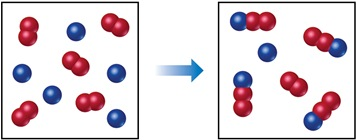# Problem: The following diagram represents a reaction shown going to completion. Each molecule in the diagram represents 0.1 mol, and the volume of the box is 1.0 L.Calculate the value of Kc.

###### FREE Expert Solution

Since we’re given the concentration of each compound, we use Kc

The Kc expression for the reaction is:

85% (316 ratings)###### Problem Details
The following diagram represents a reaction shown going to completion. Each molecule in the diagram represents 0.1 mol, and the volume of the box is 1.0 L.Calculate the value of Kc.